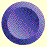Kepler's three laws provide a convenient and accurate way of describing the orbits of planets. They do not, however, give any physical reason why planetary motions obey these laws. Newton's three laws of motion, coupled with his law of gravitation, provided the reason. When Newton published his Principia in 1687, he layed the foundations of celestial mechanics. In this, the final part of the celestial sphere course, we will summarize Newton's laws and then look at how they can be used to derive Kepler's laws and predict the motion of celestial bodies.newton's laws of motionnewton's law of gravitationnewton's derivation of kepler's lawsorbital motion